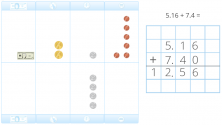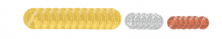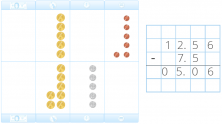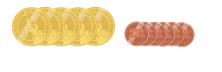Smartick is a fun way to learn math!Aug05

# Adding and Subtracting Decimal Numbers Using Money as an Example

To put the addition and subtraction of decimal numbers into practice with money, it is essential to have previous knowledge about decimals and their representation with money. Once we have mastered this knowledge, it’s time to start solving problems.

## Steps to add and subtract decimal numbers:

1. Place the numbers in columns in such a way that the ones coincide with the ones, the tens with the tens, etc.
2. Add or subtract as if they were whole numbers.
3. Finally, place the decimal (the period) in the result, separating the whole part from the decimal.

When we use money on a daily basis, we should remember that money is made up of bills which correspond to the whole part, and cents which make up the decimal.

### Example of addition with decimal numbers

This summer I want to go on a beach vacation. To get from my city to Narragansett Beach it costs $5.16 and to visit Water Wizz Water Park it costs$7.40. How much am I going to spend if I decide to go to the beach one day and the park the next?

In order to complete this problem, we begin by sorting the coins according to their amounts on the table and following the steps listed above.After completing the problem we get the sum – $12.56. The result will be represented by twelve$1 coins, five 10¢ coins, and six 1¢ coins:### Example of subtraction with decimal numbers

Otto has $12.56 that he has saved throughout the month for his first pet. When he arrives at the store he decides to buy a small hamster that costs$7.50. How much money will he have left over after the purchase?

We represent this subtraction problem in the following way:We have obtained $5.06 as a result. Therefore we will have five$1 coins and six 1¢ coins:There are many different coins and bills. Instead of using five $1 coins, we could use one$5 bill and the result would still be the same.